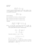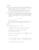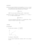MA158exam98pdfLoading previews...
 differential equations, first and second order Text Download (29kB)differential equations, first and second orderlinear system of ODEs as a matrix problemvectors and equations of planesdouble integration, plane polar coordinatestriple integration and sketching regions
6 files in this resource

MA158exam98pdf

Exam questions and solutions in PDFView Item